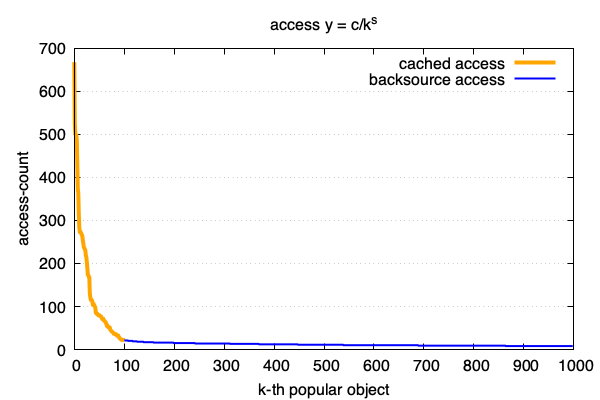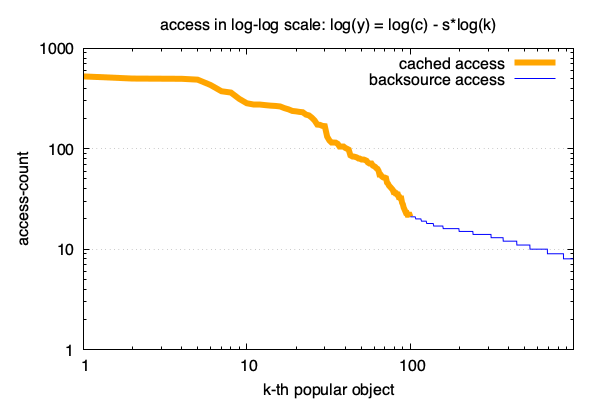# 互联网中对象访问频率的91分布

4 分钟阅读

90%的流量由10%的内容产生.$f(k) = c/k^s$

$log(f(k)) = log(c) - s \times log(k)$# 推倒

$\int_{0}^{pk}f(x) = (1-p)\int_{0}^{k}f(x)$

$p f(pk) = (1-p)f(k)$

$k_i = k_0 p^{i-1} \\ y_i = y_0 (\frac{1-p}{p})^{i-1}$

$\lg y_i = \frac {\lg \frac{1-p}{p}} {\lg p} \lg k_i + (\lg y_0 - \frac{\lg \frac{1-p}{p}}{\lg p} \lg k_0)$

# 如何将访问计数转换成对应的zipf分布的公式

python2 find-zipf.py
6.796073 - 0.708331x


$log(f(k)) = 6.796073 - 0.708331 \times log(k)$

$f(k) = \frac {894} {k^{0.708331}}$

# 标准定义

zipf在标准定义中用全量的相对占比来描述一个对象的访问频率:

Zipf’s law then predicts that out of a population of N elements, the normalized frequency of elements of rank k, f(k;s,N), is:

$f(k;s,N)={\frac {1/k^{s}}{\sum \limits_{n=1}^{N}(1/n^{s})}}$

# 有什么用

• 合理配置缓存容量以使缓存成本和性能之间做一个准确的把控.
• 在大量日志分析时, 通过合理近似降低计算量.
• 多了解一些东西. 说不定什么时候就用上了.

Discover, Design, Distribute

• Discover: 学习和探索, 找到新的东西;
• Design: 如果发现了什么新的东西, 就把它设计出来和实现出来;
• Distribute: 如果实现了什么新的东西, 那就把它带给其他人, 去让他们的生活变得更好.